IFT Notes for Level I CFA® Program
IFT Notes for Level I CFA® Program

# Part 3

## 6.  Earnings per Share

### 6.1.     Simple versus Complex Capital Structure

Earnings per share (EPS) is a very important profitability measure. It depicts the earnings per ordinary share. Some basic terminologies related to EPS are:

• Potentially dilutive securities: Securities that can be converted into ordinary shares are called potentially dilutive securities. This includes convertible bonds, convertible preferred stock, and employee stock options.
• Simple capital structure: If a company has no potentially dilutive securities it is said to have a simple capital structure.
• Complex capital structure: If a company has potentially dilutive securities it is said to have a complex capital structure.
• Dilutive securities: A potentially dilutive security that decreases EPS when exercised is called a dilutive security.
• Antidilutive security: A potentially dilutive security that increases EPS when exercised is called an antidilutive security.

### 6.2.     Basic EPS

In this calculation we do not consider the effect of any potentially dilutive securities. Basic EPS is calculated as: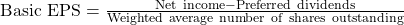Weighted average number of shares outstanding is the number of shares outstanding during the year, weighted by the portion of the year they were outstanding. Stock splits and stock dividends are applied retroactively to the beginning of the year, so the old shares are converted to new shares for consistency.

Example

During 2018, Company ABC had a net income of $100,000. It paid$22,000 as dividends to its preference shareholders and $12,000 as dividends to its common shareholders. The number of common shares outstanding during 2018 was as follows: Shares as of January 1, 2018: 10,000 Additional shares issue on July 1, 20181: 2,000 Calculate the basic EPS of the company for 2018. Solution: We had 10,000 shares outstanding for the first 6 months and 12,000 shares outstanding for the last 6 months. Therefore weighted average number of shares outstanding = 10,000 x 6/12 + 12,000 x 6/12 = 11,000 shares.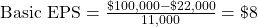Note: We ignore dividend paid to common shareholders. ### 6.3. Diluted EPS In this calculation we consider the effect of potentially dilutive securities. If a firm has a complex capital structure it has to report both basic and diluted EPS. Diluted EPS is calculated as: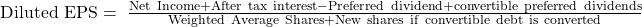For preference shares, we need to subtract preference share dividends from the numerator and add new shares issued from conversion to the denominator. Example During 2018, Company ABC had a net income of$100,000. It paid $22,000 dividends to its preference shareholders and$12,000 dividends to its common shareholders. It had 2,200 preference share and 11,000 common shares outstanding during 2018. Each preference share is convertible into 2 shares of common stock. Calculate the diluted EPS for the company.

Solution:

Number of common shares issued upon conversion = 2,200 x 2 = 4,400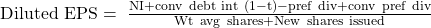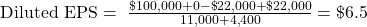For convertible bonds, we need to add the after tax interest cost savings to the numerator and new shares issued from conversion to the denominator.

Example

During 2018, Company ABC had a net income of $100,000. The capital structure of the company for 2018 was as follows: 11,000 common shares 1,000 convertible bonds with par value of$100 and 10% coupon; convertible to 5,000 shares

The tax rate of the company is 30%.

Calculate diluted EPS.

Solution:

Number of common shares issued upon conversion = 5,000

Interest payable on the bonds = 100 x $1,000 x 10% =$10,000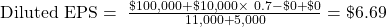For stock options, we use the ‘Treasury Stock Method’, which assumes that the hypothetical funds received by the company from the exercise of options are used to purchase shares of the company’s common stock at the average market price over the reporting period. Thus, the numerator is unchanged and the number of shares to be added to the denominator = the number of shares created by exercising the options – number of shares hypothetically repurchased with the proceeds of the exercise.

Example

During 2001, Company ABC had a net income of $100,000. It paid$22,000 dividends to its preference shareholders and $12,000 dividends to its common shareholders. The capital structure of the company for 2001 was as follows: 11,000 common shares 1,000 stock options outstanding, that have an exercise price of$20.

During 2001, the average market price for the company’s share was $25. Calculate the diluted EPS. Solution: Number of common shares issued upon conversion = 1,000 Cash proceeds from the exercise of options = 1,000 x 20 =$20,000

Number of shares that can be purchased at the average market price with these funds = \$20,000/25 = 800

Net increase in common shares outstanding = 1,000 – 800 = 200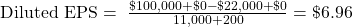Instructor’s Note:

Assess each instrument individually and determine if it is dilutive or not. Only instruments which are dilutive must be included in the diluted EPS calculation.

6.4 Changes in EPS

In general, an EPS can increase either due to an increase in net income, a decrease in the number of shares outstanding, or a combination of both.